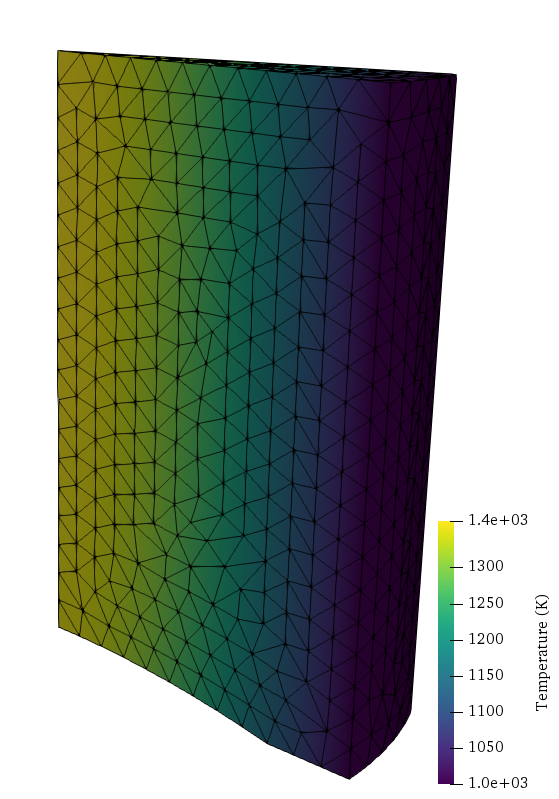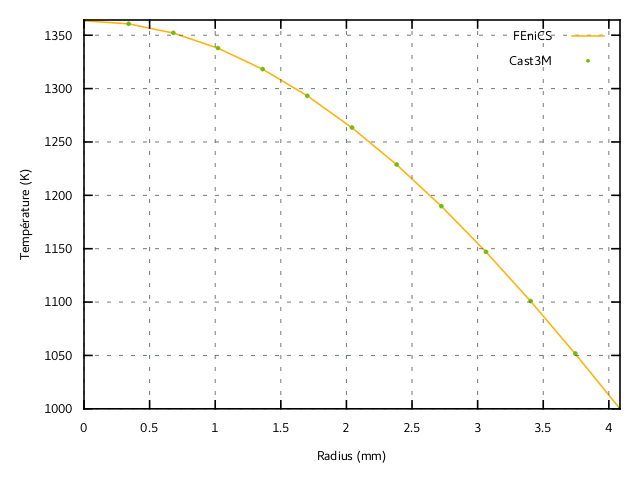trueThis example is a direct continuation of the previous 2D example on non-linear heat transfer. The present computations will use the same behaviour StationaryHeatTransfer.mfront which will be loaded with a "3d" hypothesis (default case).

Source files:

# FEniCS implementation

We now consider a portion of nuclear fuel rod (Uranium Dioxide $$\text{UO}_2$$) subject to an external imposed temperature $$T_{ext}=1000\text{ K}$$ and uniform volumetric heat source $$r=300 \text{ MW/m}^3$$. From the steady state heat balance equation $$\operatorname{div}\mathbf{j} = r$$, the variational formulation is now:

$\begin{equation} F(\widehat{T}) = \int_\Omega \mathbf{j}(T,\nabla T)\cdot\nabla \widehat{T}\,\text{dx} + \int_\Omega r \widehat{T} \,\text{dx}=0 \quad \forall \widehat{T} \end{equation}$

which fits the general default format of a MFrontNonlinearProblem: $\begin{equation} F(\widehat{u}) = \sum_i \int_\Omega \boldsymbol{\sigma}_i(u)\cdot \mathbf{g}_i(\widehat{u})\,\text{dx} -L(\widehat{u}) =0 \quad \forall \widehat{u} \end{equation}$

where $$(\boldsymbol{\sigma}_i,\mathbf{g}_i)$$ are pairs of dual flux/gradient and here the external loading form $$L$$ is given by $$-\int_\Omega r \widehat{T} \,\text{dx}$$. Compared to the previous example, we just add this source term using the set_loading method. Here we use a quadratic interpolation for the temperature field and external temperature is imposed on the surface numbered 12. Finally, we also rely on automatic registration of the gradient and external state variables as explained in the previous demo.

from dolfin import *
import mgis.fenics as mf
from time import time

mesh = Mesh()
with XDMFFile("meshes/fuel_rod_mesh.xdmf") as infile:
mvc = MeshValueCollection("size_t", mesh, 2)
with XDMFFile("meshes/fuel_rod_mf.xdmf") as infile:
facets = cpp.mesh.MeshFunctionSizet(mesh, mvc)

V = FunctionSpace(mesh, "CG", 2)
T = Function(V, name="Temperature")
T_ = TestFunction(V)
dT = TrialFunction(V)
T0 = Constant(300.)
T.interpolate(T0)

Text = Constant(1e3)
bc = DirichletBC(V, Text, facets, 12)

r = Constant(3e8)

material = mf.MFrontNonlinearMaterial("./src/libBehaviour.so",
"StationaryHeatTransfer")
problem.set_loading(-r*T*dx)

The solve method computing time is monitored:

tic = time()
problem.solve(T.vector())
print("MFront/FEniCS solve time:", time()-tic)
Automatic registration of 'TemperatureGradient' as grad(Temperature).

Automatic registration of 'Temperature' as an external state variable.

MFront/FEniCS solve time: 53.746278047561646

The temperature field along a radial direction along the top surface has been compared with computations using Cast3M finite-element solver. Both solutions agree perfectly, as shown on Figure 1.Figure 1: Temperature profile in the mid-pellet plane. Comparison of the results obtained with FEniCS

# Performance comparison

For the purpose of performance comparison, we also implement a direct non-linear variational problem with pure UFL expressions. This is possible in the present case since the non-linear heat constitutive law is very simple. Note that we enforce the use of the same quadrature rule degree. The temperature field is also reinterpolated to its previous initial value for a fair comparison between both solution strategies.

A = Constant(material.get_parameter("A"))
B = Constant(material.get_parameter("B"))
J = derivative(F, T, dT)

T.interpolate(T0)
tic = time()
solve(F == 0, T, bc, J=J)
print("Pure FEniCS solve time:", time()-tic)
Pure FEniCS solve time: 49.15058135986328
Table 1: Performance comparisons
Mesh type Quadrature degree FEniCS/MFront Pure FEniCS
coarse 2 1.2 s 0.8 s
coarse 5 2.2 s 1.0 s
fine 2 62.8 s 58.4 s
fine 5 77.0 s 66.3 s

We can observe that both methods, relying on the same default Newton solver, yield the same total iteration counts and residual values. As regards computing time, the pure FEniCS implementation is slightly faster as expected. In Table 1, comparison has been made for a coarse (approx 4 200 cells) and a refined (approx 34 000 cells) mesh with quadrature degrees equal either to 2 or 5.

The difference is slightly larger for large quadrature degrees, however, the difference is moderate when compared to the total computing time for large scale problems.

# On the use of the correct tangent operator

Most FE software do not take into account the contribution of $$\dfrac{\partial \mathbf{j}}{\partial T}$$ to the tangent operator. One can easily test this variant by assigning dj_ddT in the MFront behaviour or change the expression of the jacobian in the pure FEniCS implementation by:

J = dot(grad(T_), -grad(dT)/(A+B*T))*dx(metadata={'quadrature_degree': quad_deg})

In the present case, using this partial tangent operator yields a convergence in 4 iterations instead of 3, giving a computational cost increase by roughly 25%.